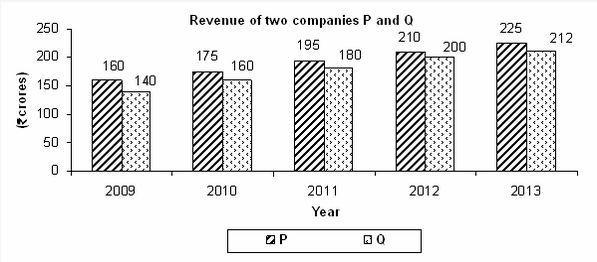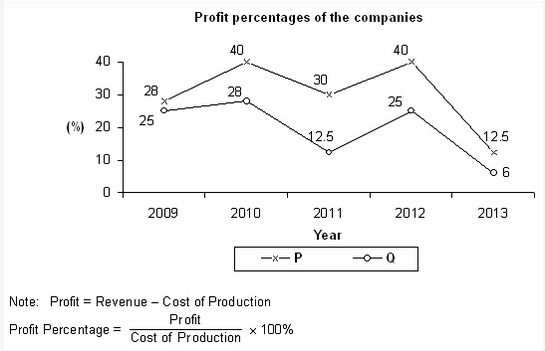#Quants Questions : Data Interpretation Set 1

Hello Aspirants. Welcome to Online Maths in AffairsCloud.com. Here we are creating question sample in Data Interpretation, which is common for all the IBPS, SBI exam and other competitive exams. We have included Some questions that are repeatedly asked in bank exams !!!1. What is the profit obtained by the two companies P and Q together in 2013?
A. Rs.33 cr
B. Rs.37 cr
C. Rs.41 cr
D. Rs.45 cr
E. None of these
[su_accordion]
B. Rs.37 cr

[/su_accordion]
2. In 2010, what was the ratio of profit of the company P to the profit of the company Q?
A. 10 : 7
B. 13 : 10
C. 16 : 13
D. 19 : 16
E.None of these
[su_accordion]
A.10 : 7

[/su_accordion]
3. What is the approximate percentage increase in the total profit of companies P and Q together from 2010 to 2012?
A. 8.45%
B. 13.35%
C. 17.65%
D. 20.25%
E. 24.15%
[su_accordion]
C. 17.65%

[/su_accordion]
4. In which of the given years was the total profit of both the companies together the highest?
A. 2009
B. 2010
C. 2011
D. 2012
E. 2013
[su_accordion]
D.2012

[/su_accordion]
5. In how many of the given years was the profit of the company P more than the profit of the company Q by at least Rs.15 crore?
A. 1
B. 2
C. 3
D. 4
E. 5
[su_accordion]
C. 3

[/su_accordion]